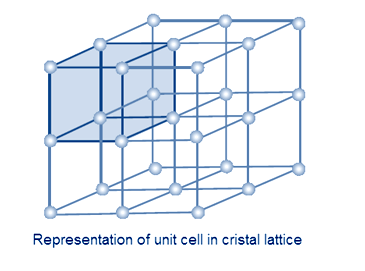# Question #bbf6e

Aug 11, 2017

WARNING! Long answer! There are four ion pairs in a unit cell of $\text{NaCl}$.

#### Explanation:

The unit cell

A unit cell is the smallest group of particles that constitutes the repeating pattern in a crystal.

Each unit cell is surrounded by other unit cells in all directions to make up a crystal lattice.The $\text{NaCl}$ unit cell

Here is a diagram of the NaCl unit cell.It is called a face centred cubic cell because the large chloride ions (green) are arranged at the faces of a cube.

The smaller sodium ions (grey) fill in the holes around the edges of the cube.

Here is an expanded view of the unit cell.You can see that there is also a sodium ion in the centre of the cube, and it is not shared with any other unit cell.

Number of $\text{Na"^"+}$ ions in unit cell

Each edge of the cube is shared by four other cubes, so there is $\frac{1}{4}$ of a sodium ion at each edge.

There are four edges at the top, four at the bottom, and four at the bottom.

$\text{Number of Na"^"+"color(white)(l) "ions" = 12 color(red)(cancel(color(black)("edges"))) × (1/4 color(white)(l)"ion")/(1 color(red)(cancel(color(black)("edge")))) + 1 color(red)(cancel(color(black)("centre")))× "1 ion"/(1 color(red)(cancel(color(black)("centre")))) = "3 ions + 1 ion" = "4 ions}$

Thus, there are four sodium ions in a unit cell of sodium chloride.

Number of $\text{Cl"^"-}$ ions in unit cell

Each corner of the cube is shared with 7 other cubes, so there is $\frac{1}{8}$ of a chloride ion at each edge.

Each face of the cube is shared by one other cube, so there is $\frac{1}{2}$ of a chloride atom at each face

$\text{Number of Cl"^"-" color(white)(l)"ions" = 8 color(red)(cancel(color(black)("corners"))) × (1/8 color(white)(l)"ion")/(1 color(red)(cancel(color(black)("corner")))) + 6 color(red)(cancel(color(black)("faces")))× (1/2color(white)(l) "ion")/(1 color(red)(cancel(color(black)("face")))) = "1 ion + 3 ions" = "4 ions}$

Thus, there are four chloride ions in a unit cell of sodium chloride.

Number of NaCl ion pairs

There are four sodium ions and four chloride ions in a unit cell, so there are four $\text{NaCl}$ ion pairs.